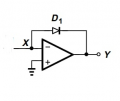# for Vin=0 how could Vy=-Vd,on ? and why is Vy is square form while Vin is sine form ?

#### Ahmet Pehlivan

Joined Oct 16, 2022
2

#### WBahn

Joined Mar 31, 2012
27,903
Notice that the case of Vin = 0 V marks a discontinuity in the claimed output waveform. So it's value exactly at Vin = 0 V is not really defined. You need to look at the case when Vin = 0- V (i.e., a small negative voltage) and Vin = 0+ V (i.e., a small positive voltage).

As for why it's a squarewave, if Vin > 0 V, what is the output Y if Vin is supplying current and the opamp is within it's active region? Now look at the waveform for Vx when Vin < 0 V. Do you understand why it is what it is? What is the output Y if Vx is anything less than 0 V?

#### Ahmet Pehlivan

Joined Oct 16, 2022
2
Notice that the case of Vin = 0 V marks a discontinuity in the claimed output waveform. So it's value exactly at Vin = 0 V is not really defined. You need to look at the case when Vin = 0- V (i.e., a small negative voltage) and Vin = 0+ V (i.e., a small positive voltage).

As for why it's a squarewave, if Vin > 0 V, what is the output Y if Vin is supplying current and the opamp is within it's active region? Now look at the waveform for Vx when Vin < 0 V. Do you understand why it is what it is? What is the output Y if Vx is anything less than 0 V?

#### ericgibbs

Joined Jan 29, 2010
16,803
hi Ahmet.
Consider the result if instead of the Sine wave source, you used a DC voltage.

So
if Vin = +1V what voltage level and polarity would the Vy be.?
Likewise
if Vin was -1V , what voltage level and polarity would the Vy be.?

E
Hint: consider the OPA Gain for both input conditions that would result by having the diode in the feedback path.

#### Jerry-Hat-Trick

Joined Aug 31, 2022
208
Maybe forget about Vin for a while, and try to think about what happens to Vy when you try to make Vx positive and when you try to make Vx negative.For working out any Op Amp problem, there are two basic rules (1) The Op Amp output always trys to make the positive and negative inputs equal (although if it's feedback loop is not connected correctly it will try but it will fail) and (2) no current flows into the Op Amp inputs. This isn't completely true, but for almost all practical purposes it is.

#### crutschow

Joined Mar 14, 2008
31,129
An op amp has very high open-loop gain so with no feedback, if the (-) input is slightly more positive than the (+) input, the output will go to the negative rail, and if it is slight more negative, the output will go to the positive rail.
If there is negative feedback, as there is here for a negative output, then the output will go to the voltage where the current through the diode matches the input signal current to keep the voltage difference between the op amp inputs very near zero.